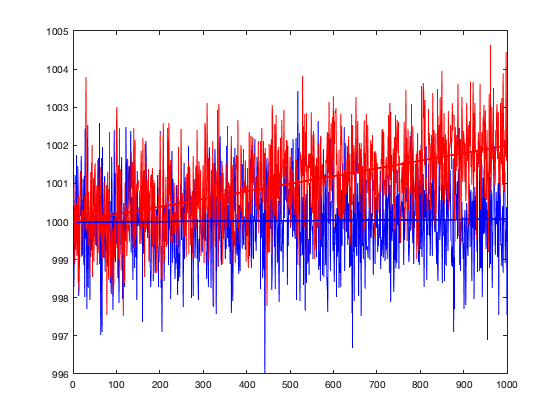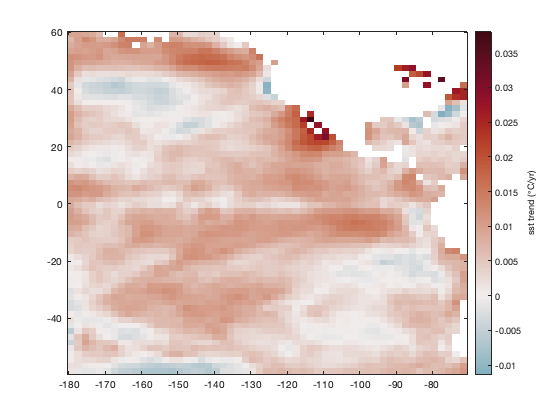# mann_kendall documentation

mann_kendall performs a standard simple Mann-Kendall test on the time series y to determine the presence of a significant trend. (Requires Statistics Toolbox)

## Syntax

```h = mann_kendall(y)
h = mann_kendall(y,alpha)
h = mann_kendall(...,'dim',dim)
[h,p] = mann_kendall(...)```

## Description

h = mann_kendall(y) performs a standard simple Mann-Kendall test on the time series y to determine the presence of a significant trend. If h is true, the trend is present; if h is false, you can reject the hypothesis of a trend. This function assumes y is equally sampled in time.

h = mann_kendall(y,alpha) specifies the alpha significance level in the range 0 to 1. Default alpha is 0.05, which corresponds to the 5% significance level.

h = mann_kendall(...,'dim',dim) specifies the dimension along which the trend is calculated. By default, if y is a 1D array, the trend is calculated along the first nonsingleton dimension of y; if y is a 2D matrix, the trend is calcaulated down the rows (dimension 1) of y; if y is a 3D matrix, the trend is calculated down dimension 3.

[h,p] = mann_kendall(...) also returns the p-value of the trend.

## Example 1: 1D array

Consider these two arrays, one with a trend and one without:

```x = (1:1000)';
y0 = randn(size(x)) + 1000;         % random data without trend
y1 = randn(size(x)) + 1000 + x/500; % random data with trend of 1/500

plot(x,y0,'b');
hold on
plot(x,y1,'r');
```To help show the trends, use polyplot, which will plot the first-degree polynomial trend lines for y0 and y1.

```polyplot(x,y0,1,'linewidth',2)
polyplot(x,y1,1,'linewidth',2)
```And just to verify the magnitudes of the trends in y0 and y1, use the trend function:

```trend(y0)
```
```ans =
-7.7958e-05
```

...as expected, the trend in y0 is about zero. Now check y1:

```trend(y1)
```
```ans =
0.0019
```

...and as expected, the trend in y1 is 1/500 as we intentionally imposed. Both of these trend magnitudes may seem like small numbers that are close to zero, while not being exactly zero. So are either of these trends significant? Use mann_kendall to find out, starting with y0:

```mann_kendall(y0)
```
```ans =
logical
0
```

The false (logical zero) confirms it: The y0 time series contains nothing but noise. Now check y1, in which, recall, we imposed a trend of 1/500:

```mann_kendall(y1)
```
```ans =
logical
1
```

The logical 1 or true answer confirms that although y1 contains noise and its trend is small in terms of magnitude, the trend is nonetheless present at the default alpha = 5% significance level. Is 5% significance not strict enough for you? Tighten it up to 0.1% like this:

```mann_kendall(y1,0.001)
```
```ans =
logical
1
```

And that confirms that the trend in y1 is present at 0.1% significance.

## Example 2: Multiple time series

If you have multiple time series in a 2D matrix, the mann_kendall function can operate on them all at once. For example, we'll make a dataset D using the two 1D array time series from Example 1:

```D = [y0 y1];
```

By default, if the input to mann_kendall is a 2D matrix, the function will operate down the rows, so testing for significance of trends in y0 and y1 is this easy:

```mann_kendall(D)
```
```ans =
1×2 logical array
0   1
```

The 0 and 1 answer means there no significant trend in the first column of D, but there is a significant trend in the second column of D.

If each time series is in its own row rather than in its own column, specify the dimension of operation like this:

```Dt = D'; % transpose D to make time go across columns of Dt.

mann_kendall(Dt,'dim',2)
```
```ans =
2×1 logical array
0
1
```

It's also possible to specify the significance level alpha. Let's really relax our standards, and set it to 99.999%:

```mann_kendall(Dt,0.99999,'dim',2)
```
```ans =
2×1 logical array
1
1
```

Wow, if we relax our standards enough, even the y0 noise contains what appears to be a significant trend!

## Example 3: 3D data

Have sea surface temperatures significantly changed in the past few decades? To answer that question, load the 60x55x802 pacific_sst dataset, which contains a grid of 802 monthly sea surface temperatures from 1950 to 2016:

```load pacific_sst
```

With a sampling rate of 12 times per year (monthly data), use the trend function to calculate the SST trend in degrees per year, and use imagescn to make a map. Set the colormap with cmocean:

```% Calculate the SST trend:
tr = trend(sst,12);

% Plot the trend:
figure
imagescn(lon,lat,tr)
cb = colorbar;
ylabel(cb,'sst trend (\circC/yr)')
cmocean('balance','pivot')
```The map above shows that in most places, the ocean seems to be warming. But is the trend significant? Use the mann_kendall function to find out, and plot the significant regions using the stipple function:

```significant = mann_kendall(sst); % (may take a second)

hold on
stipple(lon,lat,significant)
```The map above shows that the SST trend is significant most places, at the alpha = 5% level. Feel free to try it with stricter standards.

Curious about what effects the seasons might have on the calculation? Try using deseason to remove seasonal cycles from the sst dataset and re-calculate the trends and significance.

## References

Mann, H. B. (1945), Nonparametric tests against trend, Econometrica, 13, 245-259.

Kendall, M. G. (1975), Rank Correlation Methods, Griffin, London.

## Author Info

This function is part of the Climate Data Toolbox for Matlab. The function and supporting documentation were written by Chad A. Greene, adapted from the Mann_Kendall function by Simone Fatichi.